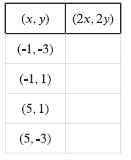# G.6.2.2 Inputs and Outputs

For each point (x, y), find its image under the transformation (x + 12, y - 2). a. A = (-10, 5) b. B = (-4, 9) c. C = (-2, 6)

Next, sketch triangle ABC and its image on the grid above. Plot points in the input bar (next to the +) by typing as a coordinate pair, ex: (-10, 5) What transformation is (x, y) (x + 12, y - 2)?

For each point (x, y) in the table, find (2x, 2y).(type answers as a coordinate pair below)

Next, sketch the original figure (the (x, y) column) and image (the (2x, 2y) column) by inputting coordinates. What transformation is (x, y)(2x, 2y)?

For a given transformation rule, what might you know about the transformation just looking at the rule?